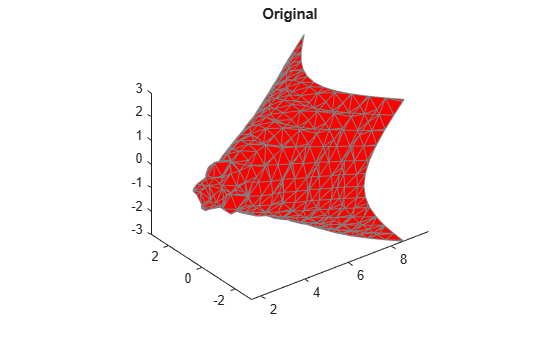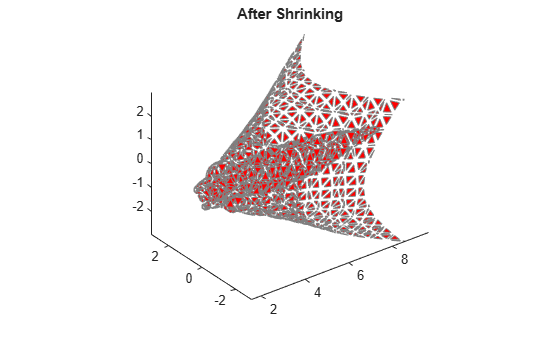# shrinkfaces

Reduce size of patch faces

## Syntax

```shrinkfaces(p,sf) nfv = shrinkfaces(p,sf) nfv = shrinkfaces(fv,sf) shrinkfaces(p) nfv = shrinkfaces(f,v,sf) [nf,nv] = shrinkfaces(...) ```

## Description

`shrinkfaces(p,sf)` shrinks the area of the faces in patch `p` to shrink factor `sf`. A shrink factor of 0.6 shrinks each face to 60% of its original area. If the patch contains shared vertices, the MATLAB® software creates nonshared vertices before performing the face-area reduction.

`nfv = shrinkfaces(p,sf)` returns the face and vertex data in the struct `nfv`, but does not set the `Faces` and `Vertices` properties of patch `p`.

`nfv = shrinkfaces(fv,sf)` uses the face and vertex data from the struct `fv`.

`shrinkfaces(p)` and `shrinkfaces(fv)` (without specifying a shrink factor) assume a shrink factor of 0.3.

`nfv = shrinkfaces(f,v,sf)` uses the face and vertex data from the arrays `f` and `v`.

`[nf,nv] = shrinkfaces(...)` returns the face and vertex data in two separate arrays instead of a struct.

## Examples

collapse all

Load the flow data set, which represents the speed profile of a submerged jet within an infinite tank. Create two isosurfaces that provide a before and after view of the effects of shrinking the face size.

Sample the flow data at every other point using `reducevolume` and then use `isosurface` to generate the faces and vertices data. Draw the first isosurface. Then set up the view and add a title.

```[x,y,z,v] = flow; [x,y,z,v] = reducevolume(x,y,z,v,2); fv = isosurface(x,y,z,v,-3); p1 = patch(fv); p1.FaceColor = 'red'; p1.EdgeColor = [0.5 0.5 0.5]; daspect([1 1 1]); view(3); axis tight title('Original')```Use `shrinkfaces` to modify the face and vertex data and draw a second patch.

```figure p2 = patch(shrinkfaces(fv,.3)); p2.FaceColor = 'red'; p2.EdgeColor = [0.5 0.5 0.5]; daspect([1 1 1]); view(3); axis tight title('After Shrinking')```## Version History

Introduced before R2006a